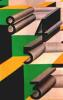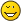Integer tetration and convergence speed rulesmarcokrtJunior FellowPosts: 29 Threads: 4 Joined: Dec 2011 12/12/2011, 12:50 AM (This post was last modified: 12/12/2011, 12:52 AM by marcokrt.) Hi all, This is my first post on this wonderful place and I have to confess I'm feeling like a kid in the playground. I've just published a quite simple book (in Italian) about integer tetration. It's mainly focused on its ending digits (perfect p-adic convergence, regular displacements of the most important constrained digits, and so on), plus some related topics. It's possible to read the first (introductive) chapter online: http://www.uni-service.it/images/stories...review.pdf The required skill level is quite low (high school proficiency), but I hope it could be a nice amusement for recreational math lovers. All the best, Marco Let $G(n)$ be a generic reverse-concatenated sequence. If $G(1) \notin \{2, 3, 7\}$, then $^{G(n)}G(n) \pmod {10^d}≡^{G({n+1})}G({n+1}) \pmod {10^d}$, $\forall n \in \mathbb{N}-\{0\}$ ("La strana coda della serie n^n^...^n", p. 60).JmsNxnUltimate FellowPosts: 1,214 Threads: 126 Joined: Dec 2010 12/12/2011, 05:20 AM (This post was last modified: 12/12/2011, 05:21 AM by JmsNxn.) Sadly, I don't speak Italian, but looking at the math that seems very wild and interesting. The fact that the same digits reoccur in $^k 3$ is very... weird? I wonder, does this happen exclusively for base ten? Would this happen in binary or hexadecimal? I think it would... right? That's very weird. This has to tell us something. Too bad I don't speak Italian to see what you've concluded. Nonetheless; this takes the cake for "mathematical beauty of the day", maybe week, for me. very cool find! I don't even know how you evaluate such large values of integer tetration of three.marcokrtJunior FellowPosts: 29 Threads: 4 Joined: Dec 2011 12/12/2011, 08:02 PM (12/12/2011, 05:20 AM)JmsNxn Wrote: Sadly, I don't speak Italian, but looking at the math that seems very wild and interesting. The fact that the same digits reoccur in $^k 3$ is very... weird? I wonder, does this happen exclusively for base ten? Would this happen in binary or hexadecimal? I think it would... right? That's very weird. This has to tell us something. Too bad I don't speak Italian to see what you've concluded. Nonetheless; this takes the cake for "mathematical beauty of the day", maybe week, for me. very cool find! I don't even know how you evaluate such large values of integer tetration of three. Thank you. Yes... it's a general outcome. BTW this represents only the beginning, I've explained every single rule concerning the convergence speed of a generic base. I've also found a lot of laws about the digits at the left of the convergent ones... To calculate this digits, it's sufficient to use Wolfram|Alpha or others free programsM Let $G(n)$ be a generic reverse-concatenated sequence. If $G(1) \notin \{2, 3, 7\}$, then $^{G(n)}G(n) \pmod {10^d}≡^{G({n+1})}G({n+1}) \pmod {10^d}$, $\forall n \in \mathbb{N}-\{0\}$ ("La strana coda della serie n^n^...^n", p. 60).nuninho1980FellowPosts: 98 Threads: 6 Joined: Apr 2009 12/20/2011, 11:29 PM (This post was last modified: 12/20/2011, 11:29 PM by nuninho1980.) I understand that.2^^3 = 16 2^^4 = 65536 2^^5 = 20035... 19718 digits ...156736 2^^6 = ???... ?.??x10^19727 digits ...8736 2^^7 = ???... ?.??x10^(?.??x10^19727) digits ...48736 4^^2 = 256 4^^3 = 13407... 145 digits ...84096 4^^4 = ???... ?.??x10^153 digits ...896 4^^5 = ???... 10^^2^153 digits ...8896 4^^6 = ???... 10^^3^153 digits ...28896marcokrtJunior FellowPosts: 29 Threads: 4 Joined: Dec 2011 12/21/2011, 12:03 AM (This post was last modified: 12/21/2011, 12:13 AM by marcokrt.) (12/20/2011, 11:29 PM)nuninho1980 Wrote: I understand that.2^^3 = 16 2^^4 = 65536 2^^5 = 20035... 19718 digits ...156736 2^^6 = ???... ?.??x10^19727 digits ...8736 2^^7 = ???... ?.??x10^(?.??x10^19727) digits ...48736 4^^2 = 256 4^^3 = 13407... 145 digits ...84096 4^^4 = ???... ?.??x10^153 digits ...896 4^^5 = ???... 10^^2^153 digits ...8896 4^^6 = ???... 10^^3^153 digits ...28896 Every base is characterized by this kind of convergence... (sometimes) there are only a few steps without convergence. The asymptotic convergence speed is constant for every base (the proof is in my book)! If you like a little more fun, you can take a look at this (in the book I have called it "sfasamento"): [5^10^i](mod 10^30): 0- 5 1- 9765625 2- 064351090230047702789306640625 3- 927874558605253696441650390625 4- 768305384553968906402587890625 5- 423444294370710849761962890625 6- 649817370809614658355712890625 7- 838703774847090244293212890625 8- 125944280065596103668212890625 9- 648495816625654697418212890625 10- 388659619726240634918212890625 11- 255141400732100009918212890625 12- 404334210790693759918212890625 13- 333762311376631259918212890625 14- 378043317236006259918212890625 15- 820853375829756259918212890625 16- 248953961767256259918212890625 … … 0- 5 1- 9765625 2- 064351090230047702789306640625 3- 927874558605253696441650390625 4- 768305384553968906402587890625 5- 423444294370710849761962890625 6- 649817370809614658355712890625 7- 838703774847090244293212890625 8- 125944280065596103668212890625 9- 648495816625654697418212890625 10- 388659619726240634918212890625 11- 255141400732100009918212890625 12- 404334210790693759918212890625 13- 333762311376631259918212890625 14- 378043317236006259918212890625 15- 820853375829756259918212890625 16- 248953961767256259918212890625 … … ... I've discovered different kinds of convergence/pseudo-convergence... it's related to caos theory too (the underlying mathematics is group theory by Galois). Marco Let $G(n)$ be a generic reverse-concatenated sequence. If $G(1) \notin \{2, 3, 7\}$, then $^{G(n)}G(n) \pmod {10^d}≡^{G({n+1})}G({n+1}) \pmod {10^d}$, $\forall n \in \mathbb{N}-\{0\}$ ("La strana coda della serie n^n^...^n", p. 60).marcokrtJunior FellowPosts: 29 Threads: 4 Joined: Dec 2011 12/21/2011, 06:21 PM We can also construct some bases with an unlimited convergence speed, for example, 999...9. The number of "9" (the lenght in digits of the base) gives us an equal "convergence speed in a single step": i.e. [9999999^^n](mod 10^(7*n))==[9999999^^(n+1)](mod 10^(7*n)). Marco Let $G(n)$ be a generic reverse-concatenated sequence. If $G(1) \notin \{2, 3, 7\}$, then $^{G(n)}G(n) \pmod {10^d}≡^{G({n+1})}G({n+1}) \pmod {10^d}$, $\forall n \in \mathbb{N}-\{0\}$ ("La strana coda della serie n^n^...^n", p. 60). « Next Oldest | Next Newest »

 Possibly Related Threads… Thread Author Replies Views Last Post Flow and convergence Daniel 3 627 03/03/2023, 01:17 PM Last Post: tommy1729 Iterated function convergence Daniel 1 550 12/18/2022, 01:40 AM Last Post: JmsNxn Frozen digits in any integer tetration marcokrt 2 745 08/14/2022, 04:51 AM Last Post: JmsNxnClosed Forms for non Integer Tetration Catullus 1 621 07/08/2022, 11:32 AM Last Post: JmsNxn The congruence speed of tetration marcokrt 2 1,630 12/31/2021, 06:58 AM Last Post: marcokrt Where is the proof of a generalized integral for integer heights? Chenjesu 2 5,791 03/03/2019, 08:55 AM Last Post: Chenjesu Tetration series for integer exponent. Can you find the pattern? marraco 20 37,438 02/21/2016, 03:27 PM Last Post: marraco Lit: f(x)=log(x) iff x is integer Gottfried 3 9,872 03/17/2015, 11:35 PM Last Post: tommy1729 Tommy's conjecture : every positive integer is the sum of at most 8 pentatope numbers tommy1729 0 4,375 08/17/2014, 09:01 PM Last Post: tommy1729 What is the convergence radius of this power series? JmsNxn 9 27,850 07/04/2011, 09:08 PM Last Post: JmsNxn

Users browsing this thread: 1 Guest(s)